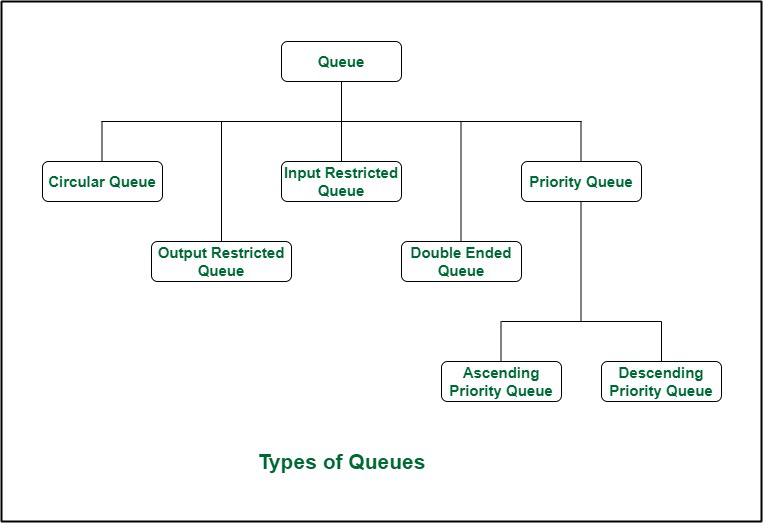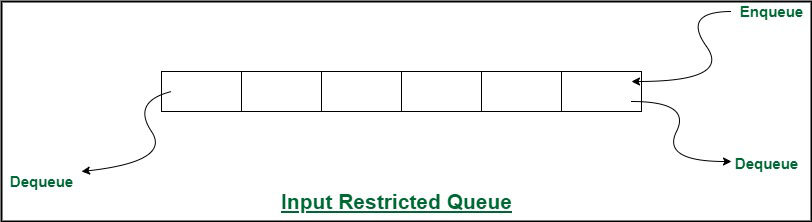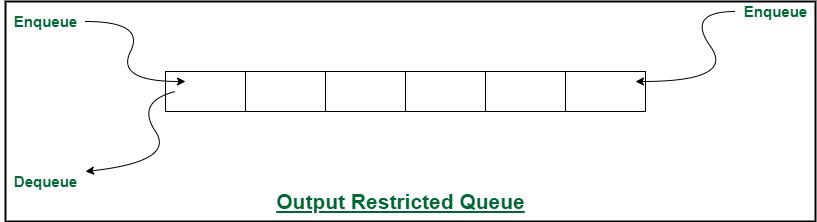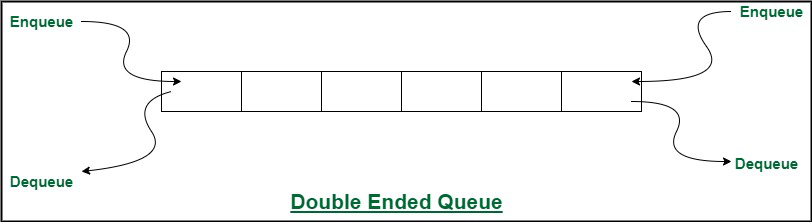# Different Types of Queues and its Applications

• Difficulty Level : Medium
• Last Updated : 23 Jun, 2022

A Queue is a linear structure that follows a particular order in which the operations are performed. The order is First In First Out (FIFO). A good example of a queue is any queue of consumers for a resource where the consumer that came first is served first. In this article, the different types of queues are discussed.

Types of Queues:

There are five different types of queues that are used in different scenarios. They are:

1. Input Restricted Queue (this is a Simple Queue)
2. Output Restricted Queue (this is also a Simple Queue)
3. Circular Queue
4. Double Ended Queue (Deque)
5. Priority Queue
• Ascending Priority Queue
• Descending Priority QueueTypes of Queues

1. Circular Queue: Circular Queue is a linear data structure in which the operations are performed based on FIFO (First In First Out) principle and the last position is connected back to the first position to make a circle. It is also called ‘Ring Buffer’. This queue is primarily used in the following cases:

1. Memory Management: The unused memory locations in the case of ordinary queues can be utilized in circular queues.
2. Traffic system: In a computer-controlled traffic system, circular queues are used to switch on the traffic lights one by one repeatedly as per the time set.
3. CPU Scheduling: Operating systems often maintain a queue of processes that are ready to execute or that are waiting for a particular event to occur.

2. Input restricted Queue: In this type of Queue, the input can be taken from one side only(rear) and deletion of elements can be done from both sides(front and rear). This kind of Queue does not follow FIFO(first in first out).  This queue is used in cases where the consumption of the data needs to be in FIFO order but if there is a need to remove the recently inserted data for some reason and one such case can be irrelevant data, performance issue, etc.Input Restricted Queue

3. Output restricted Queue: In this type of Queue, the input can be taken from both sides(rear and front) and the deletion of the element can be done from only one side(front).  This queue is used in the case where the inputs have some priority order to be executed and the input can be placed even in the first place so that it is executed first.Output Restricted Queue

4. Double ended Queue: Double Ended Queue is also a Queue data structure in which the insertion and deletion operations are performed at both the ends (front and rear). That means, we can insert at both front and rear positions and can delete from both front and rear positions.  Since Deque supports both stack and queue operations, it can be used as both. The Deque data structure supports clockwise and anticlockwise rotations in O(1) time which can be useful in certain applications. Also, the problems where elements need to be removed and or added both ends can be efficiently solved using Deque.Double Ended Queue

5. Priority Queue: A priority queue is a special type of queue in which each element is associated with a priority and is served according to its priority. There are two types of Priority Queues. They are:

1. Ascending Priority Queue: Element can be inserted arbitrarily but only smallest element can be removed. For example, suppose there is an array having elements 4, 2, 8 in the same order. So, while inserting the elements, the insertion will be in the same sequence but while deleting, the order will be 2, 4, 8.
2. Descending priority Queue: Element can be inserted arbitrarily but only the largest element can be removed first from the given Queue. For example, suppose there is an array having elements 4, 2, 8 in the same order. So, while inserting the elements, the insertion will be in the same sequence but while deleting, the order will be 8, 4, 2.

Applications of a Queue:

The queue is used when things don’t have to be processed immediately, but have to be processed in First In First Out order like Breadth First Search. This property of Queue makes it also useful in the following kind of scenarios.

1. When a resource is shared among multiple consumers. Examples include CPU scheduling, Disk Scheduling.
2. When data is transferred asynchronously (data not necessarily received at the same rate as sent) between two processes. Examples include IO Buffers, pipes, file IO, etc.
My Personal Notes arrow_drop_up
Recommended Articles
Page :